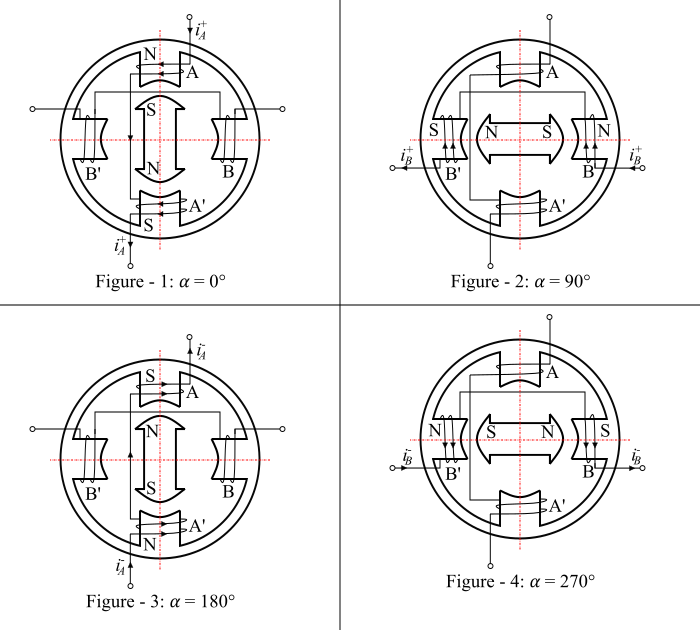# What is a Permanent Magnet Stepper Motor?

A permanent magnet (PM) stepper motor has salient pole stator. The stator has concentrated windings placed over the stator poles. It has cylindrical rotor and consists of permanent magnet poles made up of high retentivity steel. The construction of a 4/2-pole (i.e., 4-poles on the stator and 2-poles on the rotor) permanent magnet stepper motor is shown in the figure. Here, the concentrated windings on diametrically opposite poles are connected in series to form two-phase stator windings.In a permanent magnet stepper motor, the rotor poles align with the stator poles depending upon the excitation of the winding. The two coils A-A’ are connected in series to form the winding of phase A. Similarly, the two coils B-B’ are connected in series to form the winding of phase B. The stator windings A and B can be excited as follows −

• Case 1 – The current flows from the start end to the finish end of the phase winding A. This current is represented by ${{𝑖}^{+}_{𝐴}}$ and the phase winding A is denoted by A+.

• Case 2 – The current flows from the start end to the finish end of the phase winding B. This current is represented by ${{𝑖}^{+}_{B}}$ and the phase winding B is denoted by B+.

• Case 3 – The current flows from the finish end to the start end of the phase winding A. This current is represented by ${{𝑖}^{-}_{𝐴}}$ and the phase winding A is denoted by A-.

• Case 4 – The current flows from the finish end to the start end of the phase winding B. This current is represented by ${{𝑖}^{-}_{B}}$ and the phase winding B is denoted by B-.

Figure-1 represents the case, when the phase winding A is excited with current ${{𝑖}^{+}_{𝐴}}$ . In this case, the south pole of the rotor is attracted by the north pole of the stator so that the magnetic axes of the rotor and stator coincide and hence the step angle α = 0°..

Figure-2 represents the phase winding A does not carry any current and the phase winding B is energised by ${{𝑖}^{+}_{B}}$. The poles produced by the stator, now attracts the rotor poles and the rotor moves by a step of 90° in the clockwise direction and thus, the step angle is α = 90°.

Figure-3 represents the case where the phase winding A is excited by the current ${{𝑖}^{-}_{𝐴}}$ and the phase winding B does not carry any current. Hence, the rotor moves through a further step of 90° in the clockwise direction. Thus, the step angle is α = 180°.

Figure-4 represents the case when the phase winding B is excited by the current ${{𝑖}^{-}_{B}}$ and the phase winding A carries no current. The rotor again moves further by a step of 90° in clockwise direction so that the step angle is α = 270°.

For further 90° rotation of the rotor in the clockwise direction so that the step angle α = 360°,, the phase winding B is de-energised and the phase winding A is excited by the current ${{𝑖}^{+}_{𝐴}}$. Hence, four steps complete one revolution of the rotor.

From the above discussion, it is clear that in a permanent magnet stepper motor, the direction of rotation depends upon the polarity of phase currents. Therefore, for the clockwise rotor movement the sequence of exciting the stator phase windings is A+, B+, A-, B-, A+; while for anticlockwise rotation, the sequence of exciting the stator phase windings should be A+, B-, A-, B+, A+.

The permanent magnet stepper motors have high inertia and hence the lower acceleration. The maximum step rate for a permanent stepper motor is 300 pulses per second. The permanent stepper motors produce large torque per ampere stator current.

Updated on: 24-Sep-2021

761 Views